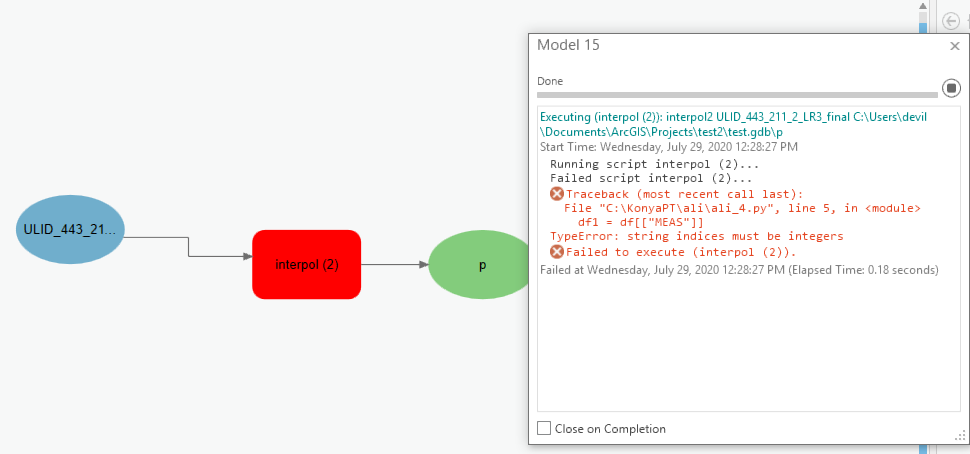# convert Pandas to Arcpy : TypeError: string indices must be integers_arcgis pro

216
1
07-29-2020 02:52 AMNew Contributor

I already have a Python pandas code to use interpolation to calculate the time for Null cells. The input and output of this code is Excel(this excel comes from a shape file) file. Now I want to do run this code as a part of the Model builder in Arcgis pro. I try to use Arcpy but I face this error continuously: "TypeError: string indices must be integers".In pandas, the attribute table automatically read as integer and matrix, so I easy search on two-column in data and then used interpolation to fill Null, but in arcpy it read it as String, so I cant search for any column, and I can't do anything to convert it.

as a summary: the code first read attribute table, then check"FS_TYPE", if it is equal to"STOP" then use "MEAS" and "TS" column to calculate "TS" of STOP. The code works properly in Pandas but I can't run it on Arcpy.

**This in normal code in Pandas:**

1. import pandas as pd
import numpy as np
path = "C:/Users/"
2. df = pd.read_excel(path + "ULID443OCT30_TIMESPACE.xlsx")
df1 = df[["MEAS"]]
for i, row in df.iterrows():
if row["F_TYPE"] =="STOP":
continue
else:
df1.loc[i,"TS"] = pd.to_datetime(row["TS"])
def calcSlope(ts1, ts2, meas1, meas2):
if meas2 - meas1 <= 10**-10:
result = 0
else:
result = (ts2-ts1)/(meas2-meas1)
return result
3. ......

**This is the code that I converted to Arcpy**

import arcpy

df = arcpy.GetParameterAsText (0)
df1 = df[["MEAS"]]
for i, row in df.iterrows():
if row["F_TYPE"] =="STOP":
continue
else:
df1.loc[i,"TS"] = arcpy.to_datetime(row["TS"])
def calcSlope(TS1, TS2, meas1, meas2):
if meas2 - meas1 <= 10**-10:
result = 0
else:
result = (TS2-TS1)/(meas2-meas1)
return result
...

Tags (5)
1 ReplybyMVP Honored Contributor

df is going to be a text reference not an object that you can slice on the second line.

Throw a print statement in there and see what I mean

... sort of retired...